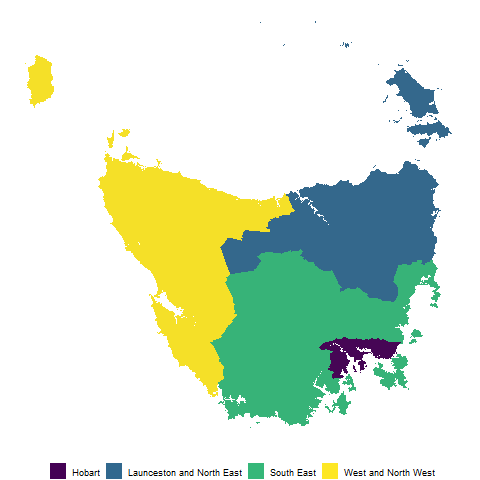# sugarbagThe sugarbag package creates tessellated hexagon maps for visualising geo-spatial data. Hexagons of equal size are positioned to best preserve relationships between individual areas and the closest focal point, and minimise distance from their actual location. This method provides an alternative to cartograms that allows all regions to be compared on the same visual scale.

Maps containing regions with a few small and densely populated areas are extremely distorted in cartograms. An example of this is a population cartogram of Australia, which distorts the map into an unrecognisable shape. The technique implemented in this package is particularly useful for these regions.

## Installation

You can install the CRAN release version of sugarbag from CRAN with:

``````# install.packages("sugarbag")
``````

You can install the development version from GitHub using:

``````# install.packages("remotes")
# remotes::install_github("srkobakian/sugarbag")
``````

## Getting started

Refer to pkgdown site: https://srkobakian.github.io/sugarbag/

``````library(sugarbag)
library(dplyr)
library(tidyr)
library(tibble)
library(ggplot2)
``````

## Creating a hexagon map of Tasmania

Tasmania is the southern-most state of Australia, it has one large land mass and several smaller islands.

### Data

We will use the Australian Bureau of Statistics’ ESRI shape files to build our map.

The set has been filtered for only Tasmanian areas. The data set of Tasmanian Statistical Areas at level two has been provided as a package data set, `?tas_sa2`.

### Centroids

The function `create_centroids` finds the central points of the polygons provided as an argument.

``````# Find the longitude and latitude centroid for each region or area
centroids <- create_centroids(tas_sa2, sf_id = "SA2_NAME16")
#> Warning in st_centroid.sf(., of_largest_polygon = largest): st_centroid assumes
#> attributes are constant over geometries of x
#> Warning in st_centroid.sfc(st_geometry(x), of_largest_polygon =
#> of_largest_polygon): st_centroid does not give correct centroids for longitude/
#> latitude data
#> Warning: st_crs<- : replacing crs does not reproject data; use st_transform for
#> that

#> Warning: st_crs<- : replacing crs does not reproject data; use st_transform for
#> that
``````

### Hexagon grid

To tessellate correctly, all the hexagons must be evenly spaced. This function creates a grid of possible locations for the polygons.

``````grid <- create_grid(centroids = centroids, hex_size = 0.2, buffer_dist = 1.2)
``````

The `sugarbag` package operates by creating a grid of possible hexagons to allocate electorates. The buffer extends the grid beyond the geographical space, this is especially useful for densely populated coastal areas or cities, such as Brisbane and Sydney in this case, Hobart.

### Allocate areas

Each polygon centroid will be allocated to the closest available hexagon grid point. The capital cities data set will be used to preserve neighbourly relationships. The `allocate` function requires two inputs, the centroids and the grid.

``````# Allocate the centroids to the hexagon grid
# We have the same amount of rows, as individual regions
hex_allocated <- allocate(centroids = centroids,
sf_id = "SA2_NAME16",
hex_grid = grid,
hex_size = 0.2, # same size used in create_grid
hex_filter = 10,
use_neighbours = tas_sa2,
focal_points = capital_cities,
# same column used in create_centroids
width = 30, verbose = TRUE)
``````

The function `fortify_hexagon` assists in plotting. We now have 6 points per region, one for each point of a hexagon. Connecting these points will allow actual hexagons to be plotted.

The additional demographic information or data can now be added. This can be used to allow plots to be coloured by region.

For animations to move between geography and hexagons the `sf_id` must match, there also needs to be an identifier to separate the states to animate between for `gganimate`.

``````hexagons <- hex_allocated %>%
fortify_hexagon(hex_size = 0.2, sf_id = "SA2_NAME16") %>%
left_join(., tas_sa2) %>%
mutate(poly_type = "hex")

polygons <- fortify_sfc(tas_sa2) %>%
mutate(poly_type = "geo")
#> Warning: The `x` argument of `as_tibble.matrix()` must have column names if `.name_repair` is omitted as of tibble 2.0.0.
#> Using compatibility `.name_repair`.
#> This warning is displayed once every 8 hours.
#> Call `lifecycle::last_warnings()` to see where this warning was generated.

ggplot(mapping = aes(fill = SA4_NAME16)) +
geom_polygon(data = polygons, aes(x=long, lat, group = interaction(SA2_NAME16,polygon)), alpha = 0.4) +
geom_polygon(data = hexagons, aes(x=long, lat, group = interaction(SA2_NAME16))) + scale_fill_viridis_d()
````````````hexagon_points <- hexagons %>%
select(SA4_NAME16, SA2_NAME16,
SA2_NAME16, long, lat, poly_type) %>%
left_join(polygons %>% distinct(SA2_NAME16, polygon), by = "SA2_NAME16")
polygon_points <- polygons %>%
select(SA4_NAME16, SA2_NAME16,
SA2_NAME16, long, lat, polygon, poly_type)
animate_tas <- bind_rows(hexagon_points, polygon_points) %>% left_join(homeless)

animate_tas %>%
ggplot(aes(x=long, y=lat, group = interaction(polygon, SA2_NAME16))) +
geom_polygon(aes(fill = SA4_NAME16)) +
geom_polygon(data = polygon_points %>% select(-poly_type), fill = "grey40", alpha = 0.05) +
coord_equal() +
theme_void() +
guides(fill = guide_legend(title = NULL)) +
theme(legend.position = "bottom") +
facet_wrap(~poly_type) +
scale_fill_viridis_d()
````````````library(gganimate)

animation <- animate_tas %>%
ggplot(aes(x=long, y=lat, group = interaction(polygon, SA2_NAME16))) +
geom_polygon(aes(fill = SA4_NAME16)) +
geom_polygon(data = polygon_points %>% select(-poly_type), fill = "grey40", alpha = 0.05) +
coord_equal() +
theme_void() +
guides(fill = guide_legend(title = NULL)) +
theme(legend.position = "bottom") +
transition_states(states = poly_type) +
scale_fill_viridis_d()

animated <- animate(animation, fps = 10, duration = 15,
start_pause = 5, end_pause = 5, rewind = FALSE)

anim_save(filename = "tasmania_animation.gif", animated)
``````## Try the sugarbag package in your browser

Any scripts or data that you put into this service are public.

sugarbag documentation built on Jan. 13, 2021, 10:11 a.m.